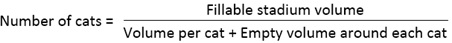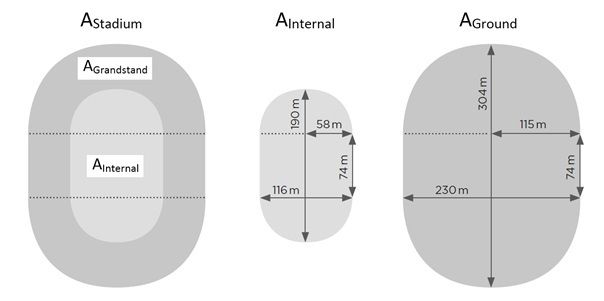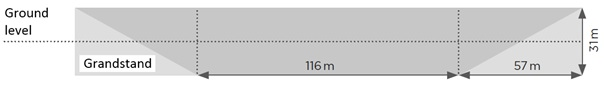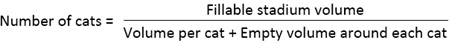# Cats in the Berlin Olympic Stadium

Topics:
Cats in the Berlin Olympic Stadium Cats in the Berlin Olympic Stadium
Rating:
3.5 (< 100 ratings)
400+
Times solved
Intermediate
Difficulty
Rating

## Problem Definition

An American marketing company plans to fill the Olympic Stadium in Berlin with cats for an advertising event and now hires your consulting company to find out how many animals they would need for this.

Some interviewers break the ice with funny or abstruse tasks. Also here, the basic principle applies to work out a comprehensible solution through valid assumptions and structured, logical procedures. Of course there are several ways of doing this. One way is presented below.

## Solution

Paragraphs highlighted in blue can be verbally communicated to the interviewee

The basic formula is the following:Thus the case can be divided into two blocks to calculate the dividend (1) and the divisor (2).

To calculate this volume, you make three simplifying assumptions:

• The upper and lower curves of the stadium are semicircles
• The area between the curves is rectangular
• The grandstands run evenly diagonally from the inner field to the upper edge

• External dimensions: 304 m in length, 230 m in width, 16 m in wall height
• Field dimensions: 190 m in length, 116 m in width, 15 m in field depth

Based on this you create a sketch:Abstraction of the stadium into simple geometric shapes

The free internal volume of the stadium can be divided into two parts:

• Volume above the inner area
• Volume above the grandstands

The area of the interior consists of two identical semicircles and a rectangle. The semicircles have a diameter of 116 m and accordingly a radius of 58 m. The semicircles have a diameter of 116 m, i.e. a radius of 58 m. The rectangle is also 116 m wide and takes 74 m of the 190 m total length. This is the part that the two semicircles do not cover with their respective 58 m. The area AInternal can accordingly be calculated as π x r2 + 1 x b = (3.14 × 582 m2 + 116 m × 74 m) = 19,147 m2. The associated height results from the 15 m of the internal area to the ground level and a further 16 m to the upper edge. It is accordingly 31 m.
VInternal is accordingly AInternal × h = 19,147 m2 × 31 m = 593,557 m3

The area of the grandstand area can be determined by subtracting the inner area from the ground area of the stadium. The latter can be calculated analogously to the first and is 58,546.5 m2. AGrandstand is accordingly AGround - AInternal = 58,546.5 m2 - 19,147 m2 = 39,399.5 m2. Multiplying this area by the height of 31 m results in the total volume above the grandstand area. This consists of the grandstand itself and the empty volume between the grandstand and the upper edge of the stadium. Because of the evenly running tribune, these two volume parts are of equal size. The free volume that can be filled with cats results accordingly from multiplying the total volume by 0.5.

VGrandstand is accordingly AGrandstand × h × 0.5 = 39,399.5 m2 × 31 m × 0.5 = 610,692.25 m3Cross-section of the stadium with grandstand and empty space

This results in a dividend of 593,557 m3 + 610,692.25 m3 = 1,204,249.25 m3.

2. Divisor: Volume per cat + Empty volume around each cat

To narrow down the task, you ask the interviewer of which kind of cats he is talking.

The interviewer replies that for safety reasons only normal domestic cats should be used (no predatory cats).

If you imagine a standing house cat with horizontally stretched head and stretched tail, this can be divided into three geometrical forms:

• 1 big cylinder (body including head)
• 4 small cylinders (legs)
• 1 small and longer cylinder (tail)

The length of the big cylinder can thus be assumed to be 35 cm. If you estimate the width of a cat's body at 10 cm, then the cylinder has a base radius of 5 cm.
VBody incl. head is accordingly π × r2 × h = 3,14 × 52 cm2 × 35 cm = 2.747,5 cm3.

For each leg, a length of 10 cm and a diameter of 2 cm can be assumed.
VLeg is accordingly π × r2 × h = 3.14 × 12 cm2 × 10 cm = 31.4 cm3

For simplification, it is now advisable to assume the same diameter for the tail as for the legs, although one can assume a length of 25 cm.
VTail is accordingly VLeg × 2,5 = 31,4 cm3 × 2,5 = 78,5 cm3

Accordingly the cat volume is:
VBody incl. head + 4 x VLeg + VTail = 2.747,5 cm3 + 4 x 31,4 cm3 + 78,5 cm3 = 2.951,6 cm3

To check your calculation, you note that a domestic cat weighs about 3 to 4 kg and its body largely consists of water (similar to the human body). Water weighs 1 kg per litre. Thus, the calculated volume of approximately 3 litres per cat seems plausible. However, due to their shape cats cannot be placed as close together as for example bricks. Therefore the volume between the stacked cats has to be considered. For reasons of time, you proceed pragmatically, increase the volume per cat by 20 percent and round off. This results in a value of 3.5 dm3 for the divisor.

Result= 1,204,249.25 m3 / 3.5 dm3 ≈ 344.071.214 cats

To fill the Berlin Olympic Stadium, 344.1 million cats are needed. For illustration: This corresponds approximately to the population of the USA.

This case was firstly published in Perspektive Unternehmensberatung 2017 by e-fellows

Do you have questions on this case? Ask our community!
400+
Times solved
Intermediate
Difficulty
Do you have questions on this case? Ask our community!